# Zipline¶

Zipline is an open-source algorithmic trading simulator.

The source can be found at: https://github.com/quantopian/zipline

Some benefits include:

• Realistic: slippage, transaction costs, order delays.
• Stream-based: Process each event individually, avoids look-ahead bias.
• Batteries included: Common transforms (moving average) as well as common risk calculations (Sharpe).
In :
import pandas as pd
import pandas.io.data
import numpy as np

import pytz
from datetime import datetime

import zipline as zp

from zipline.finance.slippage import FixedSlippage


## Simplest Zipline Algorithm: Buy Apple¶

Load Apple (AAPL) historical prices from yahoo finance.

In :
start = datetime(1990, 1, 1, 0, 0, 0, 0, pytz.utc)
end = datetime(2002, 1, 1, 0, 0, 0, 0, pytz.utc)
data.plot()

AAPL

Out:
<matplotlib.axes.AxesSubplot at 0xbbbd7ec>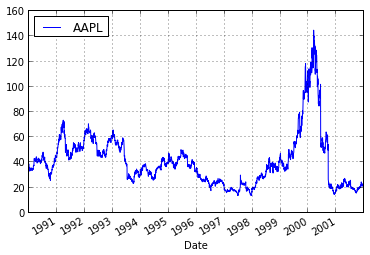Define trading algorithm by inheriting from zipline.TradingAlgorithm.

We have to overwrite two methods:

• initialize(): Called once before the beginning of the simulation
• handle_data(data): Called for every bar. Data contains only information available at this point in time.

Some commonly used attributes and methods include:

• self.order(stock, amount): Order amount of stock's shares
• self.run(data): Start the simulation. data can be a pandas.DataFrame that will be streamed through the algorithm and accessed there. It returns a DataFrame containing performance information of our algorithm.
In :
class BuyApple(zp.TradingAlgorithm): # inherit from TradingAlgorithm
def handle_data(self, data): # overload handle_data() method
self.order('AAPL', 1) # stock (='AAPL') to order and amount (=1 shares)


Instantiate class and call the .run() method to start the backtest.

In :
algo = BuyApple()
perf = algo.run(data)

[2013-07-27 13:22] INFO: Performance: Simulated 3028 trading days out of 3028.
[2013-07-27 13:22] INFO: Performance: first open: 1990-01-02 14:31:00+00:00
[2013-07-27 13:22] INFO: Performance: last close: 2001-12-31 21:00:00+00:00

In :
perf

Out:
<class 'pandas.core.frame.DataFrame'>
DatetimeIndex: 3028 entries, 1990-01-02 21:00:00 to 2001-12-31 21:00:00
Data columns (total 16 columns):
capital_used               3028  non-null values
cumulative_capital_used    3028  non-null values
ending_cash                3028  non-null values
ending_value               3028  non-null values
max_capital_used           3028  non-null values
max_leverage               3028  non-null values
orders                     3028  non-null values
period_close               3028  non-null values
period_open                3028  non-null values
pnl                        3028  non-null values
portfolio_value            3028  non-null values
positions                  3028  non-null values
returns                    3028  non-null values
starting_cash              3028  non-null values
starting_value             3028  non-null values
transactions               3028  non-null values
dtypes: float64(11), object(5)

In :
perf.portfolio_value.plot()

Out:
<matplotlib.axes.AxesSubplot at 0xeeb1d2c>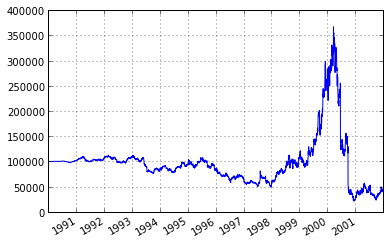• Momentum Strategy: Stocks have momentum (i.e. upward or downward trends) that we can detect and exploit.
• Mean-reversion Strategy: Stocks return to their mean and we can exploit when it deviates from that mean.

## Classic Momentum Strategy: Dual Moving Average Cross-Over¶

In :
class DualMovingAverage(zp.TradingAlgorithm):
"""Dual Moving Average Crossover algorithm.

This algorithm buys apple once its short moving average crosses
its long moving average (indicating upwards momentum) and sells
its shares once the averages cross again (indicating downwards
momentum).

"""
def initialize(self, short_window=100, long_window=400):
# Add 2 mavg transforms, one with a long window, one
# with a short window.
window_length=short_window)

window_length=long_window)

# To keep track of whether we invested in the stock or not
self.invested = False

def handle_data(self, data):
short_mavg = data['AAPL'].short_mavg['price']
long_mavg = data['AAPL'].long_mavg['price']
sell = False

if short_mavg > long_mavg and not self.invested:
self.order('AAPL', 100)
self.invested = True
elif short_mavg < long_mavg and self.invested:
self.order('AAPL', -100)
self.invested = False
sell = True

self.record(short_mavg=short_mavg,
long_mavg=long_mavg,
sell=sell)

In :
dma = DualMovingAverage()
perf = dma.run(data)

[2013-07-27 13:22] DEBUG: Transform: Running StatefulTransform [short_mavg]
[2013-07-27 13:22] DEBUG: Transform: Running StatefulTransform [long_mavg]
[2013-07-27 13:23] DEBUG: Transform: Finished StatefulTransform [long_mavg]
[2013-07-27 13:23] DEBUG: Transform: Finished StatefulTransform [short_mavg]
[2013-07-27 13:23] INFO: Performance: Simulated 3028 trading days out of 3028.
[2013-07-27 13:23] INFO: Performance: first open: 1990-01-02 14:31:00+00:00
[2013-07-27 13:23] INFO: Performance: last close: 2001-12-31 21:00:00+00:00

In :
fig = plt.figure()
ax1 = fig.add_subplot(211,  ylabel='Price in $') data['AAPL'].plot(ax=ax1, color='r', lw=2.) perf[['short_mavg', 'long_mavg']].plot(ax=ax1, lw=2.) ax1.plot(perf.ix[perf.buy].index, perf.short_mavg[perf.buy], '^', markersize=10, color='m') ax1.plot(perf.ix[perf.sell].index, perf.short_mavg[perf.sell], 'v', markersize=10, color='k') ax2 = fig.add_subplot(212, ylabel='Portfolio value in$')
perf.portfolio_value.plot(ax=ax2, lw=2.)

'^', markersize=10, color='m')
ax2.plot(perf.ix[perf.sell].index, perf.portfolio_value[perf.sell],
'v', markersize=10, color='k')

plt.legend(loc=0)
plt.gcf().set_size_inches(14, 10)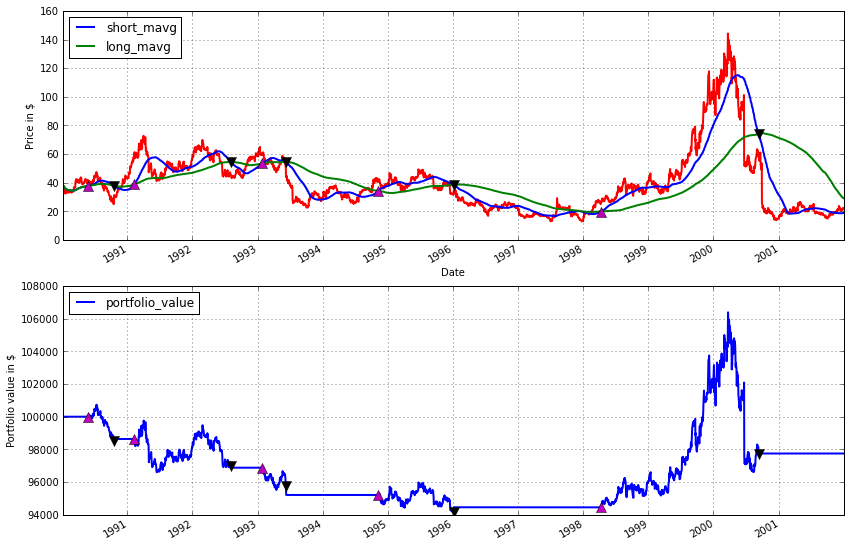## Mean-reversion strategies¶

Idea: If stock (or combination of stocks as in pairtrading) always revert back to its mean, we can long (short) the stock if it decreases (increases) from its mean.

Load stocks that are correlated (and hopefully cointegrated, which means that a linear combination of them will be mean-reverting).

Coca-Cola (KO) and Pepsi (PEP) is a good example as they are both in the same market segment and are both likely to be affected by the same events (e.g. increase of price of corn syrup).

In :
start = datetime(1997, 1, 1, 0, 0, 0, 0, pytz.utc)
end = datetime(1998, 6, 1, 0, 0, 0, 0, pytz.utc)

PEP
KO


The idea is that while neither stock price is mean-reverting, a linear combination of them will be.

In :
data[['PEP', 'KO']].plot()
plt.ylabel('price')

plt.figure()
integrated = data.PEP - data.KO
integrated.plot()
plt.ylabel('combined price')
plt.axhline(integrated.mean())

Out:
<matplotlib.lines.Line2D at 0xeb3fe6c>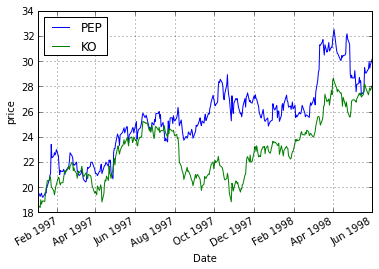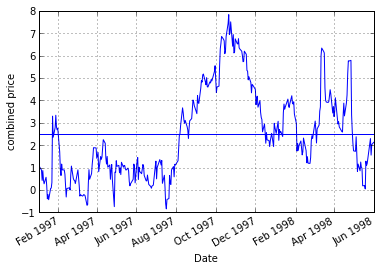In :
plt.plot(data.PEP, data.KO, 'x')
plt.xlabel('PEP')
plt.ylabel('KO')

Out:
<matplotlib.text.Text at 0xdc2898c>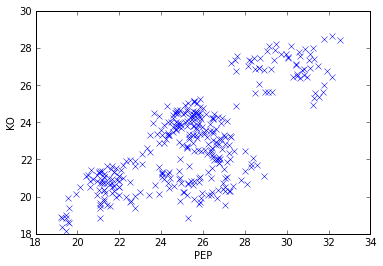Normally, we'd run a statistical test for cointegration like the Dickey-Fuller test but we'll skip this here.

## Idea of the strategy¶

Now, we can exploit this mean-reversion property of the integrated series as follows: Assume that PEP and KO drift apart, as they do Nov 1997. If we assume that they are mean-reverting we expect this gap to close again as the stocks prices come back together (which they do by Jan 1998). In other words, during this time where the spread is large, we think that PEP is overvalued, while KO is undervalued. We thus short PEP with the expectation that it will go down, and long KO under the expectation that it will go up.

## Estimating the regression coefficients¶

In this particular example it seems we can just subtract them but in reality it might be that the correlation is not 1. In our algorithm we thus estimate the regression between them in order to create a mean-reverting series using scipy.stats.linregress(price1, price2).

## BatchTransform¶

However, Zipline is designed in such a way that we ever only have access to the current price. We thus need a window of past events. The batch_transform gives us this functionality. It is a decorator for a function which will, behind the scenes, aggregate the data and pass a pandas Panel (a 3D DataFrame) into our a function.

In :
# Warning: This algorithm is written to illustrate the core idea of pairtrading.
# In reality, however, there are many more details we have to consider to make
# this actually work.

from zipline.transforms import batch_transform
from scipy import stats

@batch_transform
def regression_transform(data):
"""Computes regression coefficient (slope and intercept)
via Ordinary Least Squares between two SIDs.
"""
# data is now an array containg all past events up until now
pep_price = data.price['PEP']
ko_price = data.price['KO']
slope, intercept, _, _, _ = stats.linregress(pep_price, ko_price)

return intercept, slope

"""Pairtrading relies on cointegration of two stocks.

The expectation is that once the two stocks drifted apart
(i.e. there is spread), they will eventually revert again. Thus,
if we short the upward drifting stock and long the downward
widened we can sell the spread with profit once they converged
again. A nice property of this algorithm is that we enter the
market in a neutral position.

This specific algorithm tries to exploit the cointegration of
Pepsi and Coca Cola by estimating the correlation between the
two. Divergence of the spread is evaluated by z-scoring.
"""

def initialize(self, window_length=100):
self.set_slippage(FixedSlippage())
self.invested = False
self.regression_transform = regression_transform(refresh_period=500,
window_length=window_length)

def handle_data(self, data):
# 1. Compute regression coefficients between PEP and KO
params = self.regression_transform.handle_data(data)
if params is None:
return
intercept, slope = params

spread = (slope * data['PEP'].price + intercept) - data['KO'].price

# 3. Place orders
if spread >= 5.0 and not self.invested:
# Stocks drifted apart, long one, short the other
self.order('KO', 100)
self.order('PEP', -100)
self.invested = True
elif spread <= 2. and self.invested:
# The spread is closing again, exit positions.
# ???
# Profit!
self.order('KO', -100)
self.order('PEP', 100)
self.invested = False

# Record spread for later analysis

In :
# Run strategy

[2013-07-27 13:23] INFO: Performance: Simulated 356 trading days out of 356.
[2013-07-27 13:23] INFO: Performance: first open: 1997-01-02 14:31:00+00:00
[2013-07-27 13:23] INFO: Performance: last close: 1998-06-01 20:00:00+00:00

In :
ax1 = plt.subplot(311)
data[['PEP', 'KO']].plot(ax=ax1)
plt.ylabel('price')
plt.setp(ax1.get_xticklabels(), visible=False)

ax2 = plt.subplot(312, sharex=ax1)
ax2.axhline(2, color='k')
ax2.axhline(5, color='k')
plt.setp(ax2.get_xticklabels(), visible=False)

ax3 = plt.subplot(313, sharex=ax1)
results.portfolio_value.plot(ax=ax3, color='k')
plt.ylabel('portfolio value')

# Plot spread enter and exit markers
for ax in [ax1, ax2, ax3]:
ax.axvline(enter_pos, c='g')
ax.axvline(exit_pos, c='r')
plt.gcf().set_size_inches(16, 12)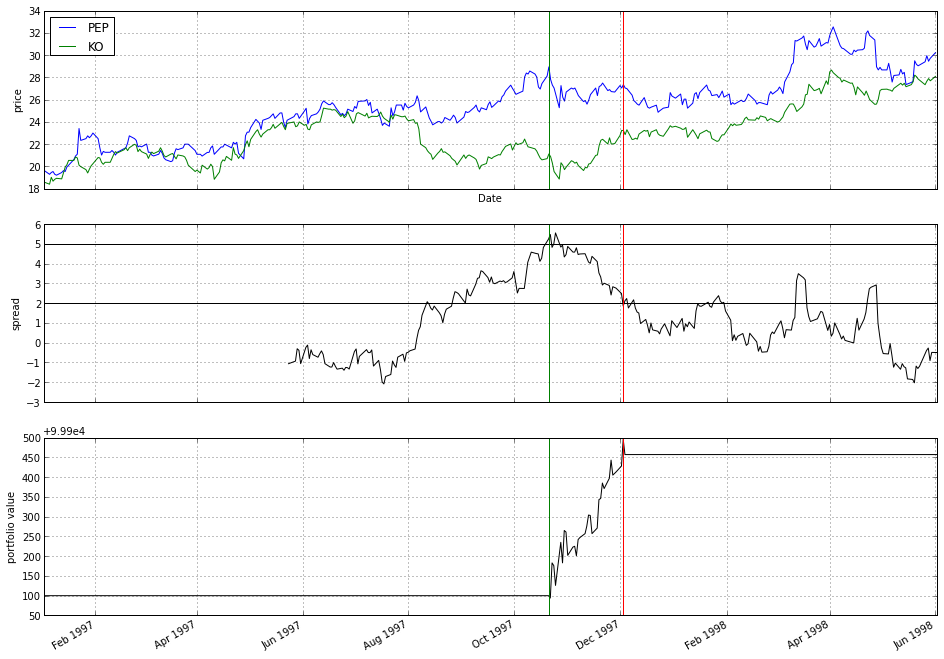## Optional: Zipline version of Google Strategy¶

In :
# Load Preis data
data.index = data.index.tz_localize(pytz.UTC)

Out:
djia debt
Date
2004-01-14 00:00:00+00:00 10485.18 0.210000
2004-01-22 00:00:00+00:00 10528.66 0.210000
2004-01-28 00:00:00+00:00 10702.51 0.210000
2004-02-04 00:00:00+00:00 10499.18 0.213333
2004-02-11 00:00:00+00:00 10579.03 0.200000
In :
# window length of 3 as in original publication
delta_t = 3

def initialize(self):
# Moving window. Defining maxlen causes the window length
# to remain constant as old events get dropped once full.
self.window = deque(maxlen=delta_t)
# Turns off slippage to simplify simulation.
self.set_slippage(FixedSlippage())

def handle_data(self, data):
window_full = len(self.window) == delta_t

# Extract debt search volume.
# 'price' is the default field used if no further information is supplied.
debt = data['debt']['price']

# Exit any prior positions
if 'djia' in self.portfolio.positions:
self.order('djia', -self.portfolio.positions['djia']['amount'])

# Moving average cross-over logic
if debt > np.mean(self.window) and window_full:
self.order('djia', -100)
elif debt < np.mean(self.window) and window_full:
self.order('djia', 100)

self.window.append(debt)